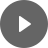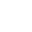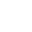4.2 Non-right Triangle - StudyNinja
Select Page

HSC Standard Maths Resources

Browse: 1. Home  »  4. Trigonometry  »  4.2 Non-right Triangle

4.2 Non-right Triangle

Non-right triangles are triangles that do not have 90-degrees inside them. For this section, make sure you are across sine rule and cosine rule.

Sine RuleThe sine rule is useful as you can use it to find either a missing angle or length of an edge of triangle if you have enough information to use the sine rule equation.

Get access to 20 Mock Exams with over 700 exam-style questions for HSC Standard Maths.

Example 1

Consider the following triangles. Find x in each of the cases:Cosine Rule

Consider the following situations:All edges of the triangle are given but no angles are provided or two edges are given and the angle formed by them are given, and the third edge is to be calculated. In situations like these, the cosine rule is the quickest way to get to the answer.

Though there are three equations below, the basic idea is the same that you put the edges and angle enclosed by those edges on right side of equation and the edge opposite the angle on the left side.Example 2

Consider the following triangles. Find x in each case:You might find the following videos helpful related to this section:4F The Sine Rule (1 of 3)

by Mr Bodgers (click to view channel)

Explains how to label a triangles vertices and edges and how this relates to the Sine Rule.4F The Sine Rule (2 of 3)

by Mr Bodgers (click to view channel)

Explains how to calculate the side on a non right-angled triangle using the sine rule.4F The Sine Rule (3 of 3)

by Mr Bodgers (click to view channel)

Explains how to calculate the size of angles on non-right angled triangles using the sine rule.4G The Cosine Rule (1 of 3)

by Mr Bodgers (click to view channel)

Explains how to label a non-right angled triangle and relates this to the Cosine Rule.4G The Cosine Rule (2 of 3)

by Mr Bodgers (click to view channel)

Completes an example where you calculate the length of a side using the Cosine Rule.4G The Cosine Rule (3 of 3)

by Mr Bodgers (click to view channel)

Completes an example where you calculate the size of an angle using the Cosine Rule.4D Trigonometry and Obtuse Angles (1 of 3)

by Mr Bodgers (click to view channel)

Explores what happens when you apply the sine, cosine and tangent function to supplementary angles.4D Trigonometry and Obtuse Angles (2 of 3)

by Mr Bodgers (click to view channel)

Completes examples where you must calculate the obtuse angle using inverse trigonometric functions.4D Trigonometry and Obtuse Angles (3 of 3)

by Mr Bodgers (click to view channel)

Completes examples where you must calculate the obtuse angle using inverse trigonometric functions.4E Area of a Triangle (1 of 2)

by Mr Bodgers (click to view channel)

Explains how to calculate the area of non-right angled triangles given the length of two sides and the included angle.4E Area of a Triangle (2 of 2)

by Mr Bodgers (click to view channel)

Explains how to calculate the area of non-right angled triangles given the length of two sides and the included angle.There are broadly 2 types of questions you can expect in exam:

Study notes of this section and other resources can be accessed here:

Browse: 1. Home  »  4. Trigonometry  »  4.2 Non-right Triangle